# KR101595772B1 - Apparatus and Method for Focusing Magnetic Field - Google Patents

## Info

Publication number
KR101595772B1
KR101595772B1 KR1020140112594A KR20140112594A KR101595772B1 KR 101595772 B1 KR101595772 B1 KR 101595772B1 KR 1020140112594 A KR1020140112594 A KR 1020140112594A KR 20140112594 A KR20140112594 A KR 20140112594A KR 101595772 B1 KR101595772 B1 KR 101595772B1
Authority
KR
South Korea
Prior art keywords
magnetic field
transmission coil
voltage
winding
plurality
Prior art date
Application number
KR1020140112594A
Other languages
Korean (ko)
Inventor
임춘택
천준필
최보환
김지훈
이귀로
김정호
조규형
김민식
조연제
김민우
Original Assignee
한국과학기술원
Priority date (The priority date is an assumption and is not a legal conclusion. Google has not performed a legal analysis and makes no representation as to the accuracy of the date listed.)
Filing date
Publication date
Application filed by 한국과학기술원 filed Critical 한국과학기술원
Priority to KR1020140112594A priority Critical patent/KR101595772B1/en
Application granted granted Critical
Publication of KR101595772B1 publication Critical patent/KR101595772B1/en

• 238000004804 winding Methods 0 claims description 83
• 239000011159 matrix materials Substances 0 claims description 47
• 238000004364 calculation methods Methods 0 claims description 10
• 230000001276 controlling effects Effects 0 claims description 5
• 238000000034 methods Methods 0 claims description 5
• 238000005516 engineering processes Methods 0 description 7
• 230000000694 effects Effects 0 description 4
• 230000001939 inductive effects Effects 0 description 2

## Images

•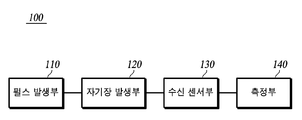•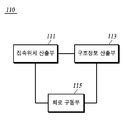•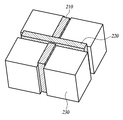•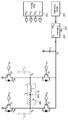••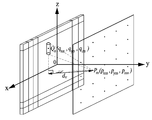•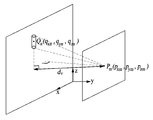•••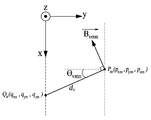•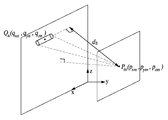•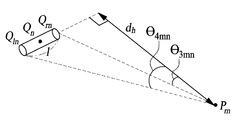•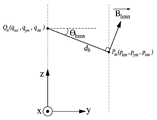•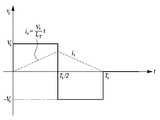•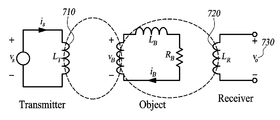•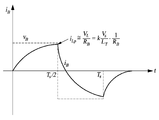••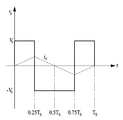## Classifications

• GPHYSICS
• G01MEASURING; TESTING
• G01RMEASURING ELECTRIC VARIABLES; MEASURING MAGNETIC VARIABLES
• G01R33/00Arrangements or instruments for measuring magnetic variables
• G01R33/20Arrangements or instruments for measuring magnetic variables involving magnetic resonance
• G01R33/28Details of apparatus provided for in groups G01R33/44 - G01R33/64
• G01R33/32Excitation or detection systems, e.g. using radio frequency signals
• HELECTRICITY
• H01BASIC ELECTRIC ELEMENTS
• H01FMAGNETS; INDUCTANCES; TRANSFORMERS; SELECTION OF MATERIALS FOR THEIR MAGNETIC PROPERTIES
• H01F5/00Coils

## Abstract

A magnetic field focusing apparatus and method are disclosed.
According to an aspect of the present embodiment, there is provided a magnetic field focusing apparatus including a plurality of transmission coils, the magnetic field focusing apparatus including: a magnetic field generating unit for focusing a magnetic field at a specific focusing point; And a voltage supply unit for supplying a voltage to the magnetic field generating unit, wherein the magnetic field generating unit includes a plurality of transmission coil modules, and the voltage supply unit independently supplies voltage to each of the plurality of transmission coil modules Magnetic field focusing device.

## Description

TECHNICAL FIELD  The present invention relates to a magnetic field focusing device,

This embodiment relates to a magnetic field focusing apparatus and method.

The contents described in this section merely provide background information on the present embodiment and do not constitute the prior art.

Magnetic Induction Tomography (MIT) uses a plurality of transmitting coils of several centimeters in size to apply a high-frequency magnetic field to an arbitrary position in a three-dimensional space, thereby generating an eddy current (magnetic induction) Eddy Current) is detected by the receiving coil.

Conventional magnetic field imaging technology has low resolution and SNR (Signal to Noise Ratio) due to mutual interference between transmitting and receiving coils and signal attenuation due to use of high frequency magnetic field. It was difficult to do.

An object of the present invention is to provide a magnetic field focusing device and method capable of focusing a magnetic field with high accuracy at an arbitrary position in three dimensions by using a highly integrated transmission coil array.

It is another object of the present invention to provide a magnetic field focusing apparatus and method having a high SNR by temporally separating signals between transmitting and receiving coils.

According to an aspect of the present embodiment, there is provided a magnetic field focusing apparatus including a plurality of transmission coils, the magnetic field focusing apparatus including: a magnetic field generating unit for focusing a magnetic field at a specific focusing point; And a voltage supply unit for supplying a voltage to the magnetic field generating unit, wherein the magnetic field generating unit includes a plurality of transmission coil modules, and the voltage supply unit independently supplies voltage to each of the plurality of transmission coil modules Magnetic field focusing device.

According to another aspect of the present invention, in the magnetic field focusing apparatus, the magnetic field focusing apparatus includes a magnetic field generating unit for focusing the magnetic field at a specific focusing point; A voltage supplier for supplying a voltage to the magnetic field generator; A receiving sensor unit generating an induced voltage by a focused magnetic field; And a measurement unit for measuring an induced voltage generated in the reception sensor unit, wherein the magnetic field generation unit includes a plurality of transmission coil modules, the reception sensor unit includes a plurality of reception coil modules, And a voltage is independently supplied to each of the transmission coil modules.

According to still another aspect of the present invention, there is provided a method of controlling a magnetic field focusing apparatus including a plurality of transmission coils, comprising the steps of independently applying voltage to each of the plurality of transmission coils, And generates a magnetic field having a specific direction and intensity at a specific position spaced apart from the magnetic field focusing device.

According to still another aspect of the present invention, there is provided a control method of a magnetic field focusing apparatus including a plurality of transmission coils and a plurality of reception coils, wherein a voltage is independently applied to each of the plurality of transmission coils, A focusing step of generating a magnetic field having a specific direction and intensity at a specific position; And measuring a shape or structure of the measurement object at the specific position by measuring an intensity of an induced voltage generated in the plurality of reception coils.

According to this embodiment, it is possible to focus the magnetic field with high precision at an arbitrary position in three dimensions using a highly integrated coil array.

In addition, according to the present embodiment, a magnetic field image having a high SNR can be obtained by temporally separating the signals between the transmitting and receiving coils.

There are various fields in which the present embodiment can be applied, and only a few of them are exemplified as follows.

When this embodiment is applied to the field of medical imaging, the inside of the human body can be observed as a high-resolution three-dimensional image of 1 mm class. Medical imaging technology using this embodiment is advantageous in that it is harmless to the human body and less expensive than MRI in comparison with X-ray.

When this embodiment is applied to the field of cancer treatment, cancer cells can be destroyed by focusing a magnetic field of several Tesla class in a local area where cancer cells are present.

When this embodiment is applied to the field of defense and counterterrorism, it is possible to observe a concrete wall, a camouflage film, a bush forest, and observe a building or an obstacle inside.

When this embodiment is applied to the field of metal detection, it is possible to quickly and accurately search underground mineral resources.

When the present embodiment is applied to an airport or a port area, the inside of baggage can be searched in place of an existing security search device using X-rays or radio waves.

By applying this embodiment to the field of distribution and logistics, it is possible to develop a new type of identification code that can replace the currently used bar code or RFID.

When the present embodiment is applied to the field of wireless power transmission, wireless power transmission with low EMF and high efficiency compared to the conventional wireless power transmission technology can be performed.

When the present embodiment is applied to a communication field, a new type of near field communication technology combining a wireless power transmission using a magnetic field focusing and a wireless communication technology can be developed.

When this embodiment is applied to the nuclear fusion field, it is possible to stably control the ions in the plasma state in the fusion reactor by using the focused magnetic field. Controlling the ions in the plasma state in the fusion reactor was one of the challenges of nuclear fusion, but it can be overcome by this embodiment.

1A is a block diagram illustrating a magnetic field focusing apparatus according to an embodiment of the present invention.
1B is a block diagram illustrating a voltage supply unit according to an embodiment of the present invention.
2 is a diagram illustrating a transmission coil module according to an embodiment of the present invention.
3 is a diagram illustrating an equivalent circuit for a circuit driver and a transmission coil module according to an embodiment of the present invention.
4 is a diagram illustrating a transmission coil array according to an embodiment of the present invention.
FIG. 5A is a diagram illustrating a direction of a magnetic field formed at a certain point on a plane parallel to a transmission coil array when a current flows in a vertical winding of a specific transmission coil module in a transmission coil array according to an embodiment of the present invention, and FIG. Fig.
Figure 5b is a simplified representation of Figure 5a.
5C is a view for explaining a magnetic field induced by a current flowing in the finite conductor.
FIG. 5D is a view of FIG. 5B in the yz plane direction.
FIG. 5E shows the view of FIG. 5B in the xy plane direction.
FIG. 6A is a diagram illustrating a direction of a magnetic field formed at a certain point on a plane parallel to a transmission coil array when a current flows in a horizontal direction winding of a specific transmission coil module in a transmission coil array according to an embodiment of the present invention, and FIG. Fig.
6B is a view of FIG. 6A in the xy plane direction.
Fig. 6C is a view of Fig. 6A in the yz plane direction.
Figure 7a is applied to the positive pulse voltage + V S to the transmission coil in the magnetic field focusing device according to one embodiment of the invention, at 0T 0.5T S by S, a negative voltage pulse to the transmit coil 1T S by S at 0.5T - And V S are applied, waveforms of currents flowing through the transmission coil by the applied voltage are shown.
7B is a diagram illustrating an equivalent circuit for the transmission coil module, the measurement object, and the reception coil module in the magnetic field imaging apparatus using the magnetic field focusing apparatus according to the embodiment of the present invention.
FIG. 7C is a diagram showing a magnetic field focusing apparatus using a magnetic field focusing apparatus according to an embodiment of the present invention. In FIG. 7C, when a voltage as shown in FIG. 7A is applied to a transmission coil, Fig. 8 is a diagram illustrating a waveform of a current to be applied.
7D is a diagram illustrating a waveform of a voltage detected by a receiving coil when a current as shown in FIG. 7C is induced in a measurement object in a magnetic field imaging apparatus using a magnetic field focusing apparatus according to an embodiment of the present invention.
7E, in the magnetic field focusing device according to the embodiment of the present invention, a positive voltage pulse + V S is applied to the transmission coil from 0T S to 0.25T S , and a negative voltage pulse is applied to the transmission coil from 0.25T S to 0.75T S -V S is applied, and from 0.75T S to 1T S , the waveform of the current flowing in the transmission coil due to the applied voltage when positive voltage pulse + V S is applied to the transmission coil.

Hereinafter, some embodiments of the present invention will be described in detail with reference to the accompanying drawings. It should be noted that, in the drawings, like reference numerals are used to denote like elements in the drawings, even if they are shown in different drawings. In the following description of the present invention, detailed description of known functions and configurations incorporated herein will be omitted when it may make the subject matter of the present disclosure rather unclear.

In describing the constituent elements of this embodiment, first, second, i), ii), a), b) and the like can be used. Such a code is intended to distinguish the constituent element from other constituent elements, and the nature of the constituent element, the order or the order of the constituent element is not limited by the code. It is also to be understood that when an element is referred to as being "comprising" or "comprising", it should be understood that it does not exclude other elements unless explicitly stated to the contrary, do. Also, the terms 'module', 'module' and the like described in the specification mean a unit for processing at least one function or operation, which can be implemented as 'hardware' or 'software' or 'combination of hardware and software' have.

The technology proposed in this embodiment is a high-resolution SMF (High-Resolution Synthesized Magnetic Field Focusing) technique, in which a controlled current is supplied to a plurality of (for example, several thousand) transmission coils by a precisely controlled voltage, It is a technology to concentrate.

According to this embodiment, even if the magnetic field focusing device is not close to the position where the magnetic field is intended to be converged but is spaced apart by a certain distance, the user can focus the magnetic field having the direction and intensity desired by the user at a desired position.

1A is a block diagram illustrating a magnetic field focusing apparatus according to an embodiment of the present invention.

The magnetic field focusing apparatus 100 according to the present embodiment includes a voltage supply unit 110, a magnetic field generation unit 120, a reception sensor unit 130, and a measurement unit 140. However, some of the components of the magnetic field focusing apparatus 100 may be omitted or other components may be added according to the embodiment.

For example, in a magnetic field focusing apparatus for focusing a magnetic field of several Tesla class at a local site where cancer cells are present in the field of cancer treatment, a desired purpose can be achieved only by the voltage supplying unit 110 and the magnetic field generating unit 120.

As another example, in a magnetic field focusing apparatus for focusing a magnetic field at a specific point where a terminal is located in the field of wireless power transmission to enable high-efficiency wireless charging, if a voltage supplying unit 110 and a magnetic field generating unit 120 are required, can do.

The magnetic field focusing device including the voltage sensor 110 and the magnetic field generator 120 but not including the receiving sensor 130 and the measuring unit 140 may be implemented as needed.

Hereinafter, each component of the magnetic field focusing apparatus 100 will be described in detail.

The magnetic field generator 120 includes a plurality of transmission coil modules. The shape, function, and arrangement of the transmission coil module constituting the magnetic field generation unit 120 will be described later in detail.

The voltage supplying unit 110 supplies a voltage to the magnetic field generating unit 120. The magnetic field generator 120 includes a transmission coil array, and the transmission coil array includes a plurality of transmission coil modules. The voltage supplier 110 is connected to each transmission coil module in the transmission coil array of the magnetic field generator 120, And the voltage is applied independently. Also, the voltages applied to the vertical direction windings and the horizontal direction windings of the respective transmission coil modules by the voltage supply unit 110 are independent of each other.

The reception sensor unit 130 includes a plurality of reception coil modules. The receiving coil module generates an induced voltage according to an induced current generated at the focusing point by a magnetic field focused on the focusing point. The reception coil module may be disposed adjacent to the transmission coil module, but is not limited thereto and may be disposed at various positions depending on the application purpose.

The measuring unit 140 measures the intensity of the induced voltage or induced current generated in the plurality of receiving coil modules.

Hereinafter, each component of the voltage supply unit 110 will be described in detail.

1B is a block diagram illustrating a voltage supply unit according to an embodiment of the present invention.

The voltage supply unit 110 according to the present embodiment includes a focusing information input unit 111, a structure information calculation unit 113, and a circuit driving unit 115.

The focus information input unit 111 receives information about a point at which a magnetic field is to be focused (hereinafter referred to as a focus point). The information on the focal point can be selected by the user from among a plurality of focal point candidates that can be set as the focal point. The focusing information input unit 111 receives information about the direction and intensity of the magnetic field to be focused on the focal point from the user.

The structure information calculation unit 113 uses the structure information according to the positional relationship between the plurality of focus point candidates and the plurality of transmission coil modules and the characteristics of the magnetic field transmission medium to focus the magnetic field input from the focus information input unit 111 The voltage to be applied to each transmit coil module is calculated. At this time, the structure information according to the positional relationship between the plurality of focal point candidates and the plurality of transmission coil modules and the characteristics of the magnetic field transmission medium may be stored in a storage medium such as a memory in a state calculated corresponding to each focal point candidate have.

The specific calculation method of the voltage to be applied to each transmission coil module depends on the form of the transmission coil array. In this embodiment, as an example of one of them, the intensity of the voltage to be applied to each transmission coil module in a case where the transmission coil array is configured in a planar form will be described later using the mathematical expression.

The circuit driver 115 applies a voltage to each transmission coil module according to the voltage value calculated by the structure information calculation unit 113. In this case, the voltage applied by the circuit driver 115 is preferably a pulse wave.

This embodiment describes the actual implementation of a high-resolution magnetic field focusing apparatus, method and magnetic field receiving apparatus therefor (Application No. 10-2013-0127402) filed by the inventor and applicant on Oct. 24, 2013 .

Although the magnetic field generating unit 120 is abstractly described in the application (Application No. 10-2013-0127402), the present embodiment discloses the specific shape and function of the magnetic field generating unit 120.

2 is a diagram illustrating a transmission coil module according to an embodiment of the present invention.

The transmission coil module is configured such that a winding 210 in the vertical direction and a winding 220 in the horizontal direction are wound around the core 230.

The shape of the core 230 is preferably a square pillar shape, and the one end face of the core 230 is preferably a square shape. If the shape of the core 230 is a quadrangular prism and one end face of the core 230 is a square shape, the length of the front portion of the winding 210 in the vertical direction wound on the core 230, The length of the front portion of the winding 220 in the direction becomes equal.

The vertical winding 210 and the horizontal winding 220 are wound around the core 230 assuming that the core 230 is in the shape of a quadrangular prism and that one end face of the core 230 is square. Calculate the intensity of the current to flow to each.

However, even if one end face of the core 230 is not a square but a rectangular shape, the direction and intensity of the magnetic field integrated at a specific position may be calculated by modifying the value of l in the following equation. For example, if one end surface of the core 230 is in the form of a rectangle rather than a square, the length of the vertical winding of the n-th transmission coil module is denoted by l 1 and the length of the horizontal winding of the n-th transmission coil module L 2 , the direction and intensity of the magnetic field integrated at a specific position can be easily calculated.

The voltage supply unit 110 applies a voltage to the vertical winding 210 and the horizontal winding 220 of the transmission coil module independently of each other. The currents flowing in the vertical winding 210 and the horizontal winding 220 are independent of each other.

3 is a diagram illustrating an equivalent circuit for a circuit driver and a transmission coil module according to an embodiment of the present invention.

3, the circuit driver 115 includes a microprocessor 240, a DC power source 250, a converter 260, a gate driver 270, a capacitor C, a first switch Q1, 2 switch Q2, a third switch Q3, a fourth switch Q4 and a transmission coil 280. [

In Figure 3, the transmit coil 280 includes a coil L c and a resistor R c . The transmission coil 280 is connected to the circuit driver 115.

In FIG. 3, when both ends of the transmission coil are referred to as nodes X and Y, V X and V Y denote voltages of nodes X and Y, respectively, and C X and C Y denote elements at nodes X and Y, Which is a parasitic capacitance value. The operation efficiency of the circuit driver 115 can be increased by performing zero voltage switching (ZVS) using the voltage values of V X and V Y in the gate driver 270.

4 is a diagram illustrating a transmission coil array according to an embodiment of the present invention.

The magnetic field generator 120 includes a transmission coil array. The transmission coil array is a module in which a plurality of transmission coil modules are combined.

The transmission coil array is configured in such a manner that a plurality of transmission coil modules are arranged horizontally and vertically on a plane.

However, according to the embodiment, the plurality of transmission coil modules may be arranged on various solid shapes such as a curved surface, a spherical surface, an elliptical surface, and a cylindrical surface. For example, the magnetic field focusing device used for imaging a medical image is preferably a configuration in which a plurality of transmission coil modules are arranged on a cylindrical surface.

A plurality of transmission coil modules are arrayed horizontally and vertically on a plane to constitute a transmission coil array. The directions and intensities of the magnetic fields generated by the currents flowing through the transmission coil modules will be described using mathematical expressions.

FIG. 5A is a diagram illustrating a direction of a magnetic field formed at a certain point on a plane parallel to a transmission coil array when a current flows in a vertical winding of a specific transmission coil module in a transmission coil array according to an embodiment of the present invention, and FIG. Fig.

Figure 5b is a simplified representation of Figure 5a.

In FIG. 5B, Q n is the center point of the n-th transmission coil module, and the three-dimensional coordinates of Q n are expressed by (q xn , q yn , q zn ).

P m is the m-th focal point, and the three-dimensional coordinates of P m are expressed as (p xm , p ym , p zm ).

A magnetic field is induced when a current flows in a vertical winding or a horizontal winding of the transmission coil module. Since the winding in the vertical direction or the winding in the horizontal direction of the transmission coil module has a finite length, the magnetic field induced by the current flowing in the vertical winding or the horizontal winding is a magnetic field induced by the current flowing in the finite conductor .

5C is a view for explaining a magnetic field induced by a current flowing in the finite conductor.

According to the Biot-Savart's Law, the intensity of the magnetic field induced by the current flowing in the finite conductor of minute length is expressed by Equation (1).

In the equation (1), dB is the magnetic flux density of the magnetic field induced by the current flowing through the micro-length finite conductor, I 0 is the permeability in free space, I is the intensity of the current flowing through the conductor,

Is a unit vector perpendicular to the finite line, r is the vertical distance from the extension of the finite line to the focal point, y 0 is the value of the y coordinate of the focal point, Is a unit vector indicating the direction of the magnetic flux.

Assuming that the finite conductor is located from z 1 to z 2 on the z-axis, the magnetic flux density of the magnetic field induced by the current flowing in the finite conductor can be obtained by integrating Equation 1 from z 1 to z 2 with respect to z. The integration result is shown in Equation (2).

B is the magnetic flux density of the magnetic field induced by the current flowing in the finite conductor of length z 2 - z 1 , θ 1 is the angle formed by the line segment connecting y 0 and z 1 with respect to the y axis, θ 2 is the angle formed by the line segment connecting y 0 and z 2 with respect to the y-axis.

As shown in Equation (2), when a current flows through the finite conductor, a magnetic field is formed around the conductor. According to the Biasarv's law, the direction and intensity of the magnetic field formed at a specific point can be determined by the current flowing through the finite conductor.

FIG. 5D is a view of FIG. 5B in the yz plane direction.

In FIG. 5D, Q un represents the coordinates of the upper end (up) of the vertical winding of the nth transmission coil module.

Q dn represents the coordinates of the lower end of the winding in the vertical direction of the nth transmission coil module.

θ 1mn is an angle formed by a line segment connecting the lower end of the vertical winding of the nth transmission coil module and the mth focusing point with respect to d v .

θ 2mn is an angle formed by a line segment connecting the upper end of the vertical winding of the nth transmission coil module and the mth focusing point with respect to d v .

l is the length of the winding in the vertical direction of the nth transmission coil module.

d v can be calculated as shown in Equation (3).

The length from Q dn to P m is shown in Equation (4).

The length from Q un to P m is given by Equation (5).

The value of sin &amp;thetas; 1mn is expressed by Equation (6).

The value of sin [theta] 2mn is given by Equation (7).

FIG. 5E shows the view of FIG. 5B in the xy plane direction.

In Figure 5e, vmn θ is, the angle d with respect to the y-axis forms v.

B vmn is a magnetic field induced at the position of the m-th focusing point by the vertical winding of the n-th transmission coil module. The direction vector of B vmn is on the xy plane.

Since B vmn is a magnetic field induced by the current flowing through the finite conductor, the intensity of B vmn when Equation (2) is applied is expressed by Equation (8).

In practice, however, the magnetic mirror effect must be considered. According to the publication "Finite-Width Magnetic Mirror Models of Mono and Dual Coils for Wireless Electric Vehicles" issued by IEEE TRANSACTIONS ON POWER ELECTRONICS, vol. 28, No. 3, MARCH 2013 published in 2013, It is known that the intensity of a magnetic field is doubled when a magnetic field is generated.

The intensity of B vmn considering the magnetic mirror effect is expressed by Equation (9).

Theta vmn can be obtained using the trigonometric function as shown in Equation (10).

As a result, the magnetic field B vmn induced at the position of the m-th focusing point by the vertical winding of the n-th transmission coil module is expressed by Equation (11).

Assuming that the number of transmission coil modules is N, the magnetic field B v induced at the position of the m-th focusing point by the vertical winding of the N transmission coil modules is expressed by Equation (12).

Since the direction vector of B vmn exists on the xy plane, when calculating B vmn , only the components in the x direction and y direction are considered.

In Equation (12), B vmn, x represents a component in the x direction of the magnetic field induced at the position of the mth focusing point by the vertical winding of the nth transmission coil module. B vmn, y represents the y-direction component of the magnetic field induced at the position of the m-th focusing point by the vertical winding of the n-th transmission coil module.

B vmn, x is expressed by Equation (13).

B vmn, y is expressed by Equation (14).

The components of the magnetic field in the x direction induced by the M winding points of the N transmission coil modules in the vertical direction can be represented by Equation (15) using a matrix.

In Equation (15), B vx is an M × 1 matrix, A vx is an M × N matrix, and I v is an N × 1 matrix.

a vmn, x represents the component in the x direction of the magnetic field induced in the position of the m-th focusing point by the vertical winding of the n-th transmission coil module to the current value I vn flowing in the vertical winding of the n-th transmission coil module It means divided value. Dividing the equation (13) by I vn can obtain a vmn, x .

a vmn, x is expressed by Equation (16).

The components in the y direction of the magnetic field induced and synthesized at the positions of M focus points by the windings in the vertical direction of the N transmission coil modules can be expressed by Equation (17) using a matrix.

In Equation 17, B vy is an M × 1 matrix, A vy is an M × N matrix, and I v is an N × 1 matrix.

a vmn, y represents the y-direction component of the magnetic field induced at the position of the m-th focal point by the vertical winding of the n-th transmission coil module to the current value I vn flowing in the vertical winding of the n-th transmission coil module It means divided value. By dividing Equation (14) by I vn , a vmn, y can be obtained.

a vmn, y is represented by Equation (18).

By combining the matrices, the component in the x direction and the component in the y direction of the magnetic field, which are induced at the positions of the M focus points by the vertical winding of the N transmit coil modules, can be represented by one matrix.

The matrix B vx of Equation (15) and the matrix B vy of Equation (17) are combined as shown in Equation (19).

In Equation 19, the synthesized matrix B v is a 2M x 1 matrix.

The matrix A vx of Equation (15) and the matrix A vy of Equation (17) are synthesized as shown in Equation (20).

In Equation 20, the synthesized matrix A v is a 2M x N matrix.

As a result, the component in the x direction and the component in the y direction of the magnetic field, which are induced and synthesized at the positions of M focus points by the windings in the vertical direction of the N transmission coil modules, can be expressed by a matrix.

Equation 21 is derived by the following procedure.
When A v + is the right inverse of A v , B v = A v (A v + ) B v holds.
B v = A v I v to v = A v B (A + v) Substituting B v A v (A v + ) B v = A v I v is derived.
Since A v (A v + ) B v = A v I v , I v = (A v + ) B v can be set. A right inverse of v in a linear algebra A v T because (A v v A T) -1, I v A = v T (A v A v T) is satisfied is -1 B v.

The right inverse of A v in linear algebra exists only if it is 'column of A v , row ≤A v '. Since the rows of A v are 2M and the columns are N, N &gt; = 2M in order to satisfy the expression (21).

For example, suppose that we want to synthesize a magnetic field of a certain intensity with the direction on the xy plane at the mth focal point. In this case, by modifying the B v matrix according to the position, direction and intensity of the magnetic field to be synthesized, and substituting it into the equation (21), a matrix I v is derived. By analyzing the derived matrix I v , we can calculate the current that flows into the vertical windings of each transmit coil module.

FIG. 6A is a diagram illustrating a direction of a magnetic field formed at a certain point on a plane parallel to a transmission coil array when a current flows in a horizontal direction winding of a specific transmission coil module in a transmission coil array according to an embodiment of the present invention, and FIG. Fig.

In FIG. 6A, Q n is the center point of the n-th transmission coil module, and the three-dimensional coordinates of Q n are represented by (q xn , q yn , q zn ).

P m is the m-th focal point, and the three-dimensional coordinates of P m are expressed as (p xm , p ym , p zm ).

6B is a view of FIG. 6A in the xy plane direction.

6B, Q ln represents the coordinate of the left end of the winding in the horizontal direction of the nth transmission coil module.

Q rn represents the coordinate of the right end of the horizontal winding of the nth transmission coil module.

θ 3mn is an angle formed by a line segment connecting the lower end of the horizontal winding of the nth transmission coil module and the mth focusing point with respect to d h .

θ 4mn is the angle formed by the line segment connecting the upper end of the horizontal winding of the nth transmission coil module and the mth focusing point with respect to d h .

l is the length of the winding in the horizontal direction of the nth transmission coil module.

d h can be calculated by Equation (22).

The length from Q rn to P m is given by Equation 23.

The length from Q ln to P m is shown in Equation 24.

The value of sin? 3mn is expressed by Equation 25.

The value of sin [theta] 4mn is expressed by Equation (26).

Fig. 6C is a view of Fig. 6A in the yz plane direction.

In Fig. 6C ,? Hmn is an angle formed by dh with respect to the y-axis.

B hmn is a magnetic field induced at the position of the m-th focusing point by the horizontal winding of the n-th transmission coil module. The direction vector of B hmn is on the yz plane.

Since B hmn is a magnetic field induced by the current flowing in the finite conductor, the intensity of B hmn when Equation (2) is applied is expressed by Equation (27).

In practice, however, the magnetic mirror effect must be considered. The intensity of B hmn considering the magnetic mirror effect is expressed by equation (28).

Theta hmn can be obtained by using the trigonometric function as shown in Equation (29).

As a result, the magnetic field B hmn induced at the position of the m-th focal point by the winding in the horizontal direction of the n-th transmission coil module is expressed by Equation (30).

Assuming that the number of transmission coil modules is N, the magnetic field B h induced at the position of the m-th focusing point by the horizontal winding of the N transmission coil modules is expressed by Equation (31).

Since the direction vector of B hmn is on the yz plane, when calculating B hmn , only the components in the y and z directions are considered.

In Equation 31, B hmn, y represents a y-direction component of the magnetic field induced at the position of the m-th focusing point by the horizontal winding of the n-th transmission coil module. B hmn, z represents the z-direction component of the magnetic field induced at the position of the m-th focusing point by the horizontal winding of the n-th transmission coil module.

B hmn, y is expressed by Equation (32).

B hmn, z is expressed by Equation (33).

The components in the y direction of the magnetic field induced and synthesized at the positions of the M focus points by the windings in the horizontal direction of the N transmission coil modules can be expressed by Equation (34) using a matrix.

In Equation 34, B hy is an M × 1 matrix, A hy is an M × N matrix, and I h is an N × 1 matrix.

a hmn, y represents the y-direction component of the magnetic field induced at the position of the m-th focusing point by the horizontal winding of the n-th transmission coil module to the current value I hn flowing in the horizontal winding of the n-th transmission coil module It means divided value. By dividing the expression (32) by I hn , a hmn, y can be obtained.

a hmn, y is expressed by Equation (35).

The component in the z direction of the magnetic field induced and synthesized at the positions of M focus points by the windings in the horizontal direction of the N transmission coil modules can be expressed by Equation 36 using a matrix.

In Equation 36, B hz is an M x 1 matrix, A hz is an M x N matrix, and I h is an N x 1 matrix.

a hmn, z represents the z-direction component of the magnetic field induced at the position of the m-th focal point by the horizontal winding of the n-th transmission coil module to the current value I hn flowing in the horizontal winding of the n-th transmission coil module It means divided value. By dividing the equation (33) by I hn , a hmn, z can be obtained.

a hmn, z is expressed by Equation (37).

When the matrix is synthesized, the components in the y direction and the components in the z direction of the magnetic field, which are induced at the positions of the M focus points by the horizontal winding of the N transmission coil modules, can be represented by one matrix.

The matrix B hy of Equation (34) and the matrix B hz of Equation (36) are combined to obtain Equation (38).

In equation (38), the synthesized matrix B h is a 2M x 1 matrix.

The matrix A hy of equation (34) and the matrix A hz of equation (36) are combined as shown in equation (39).

In Equation 39, the synthesized matrix A h is a 2M x N matrix.

As a result, the y-direction component and the z-direction component of the magnetic field induced and synthesized at the positions of M focus points by the windings in the horizontal direction of the N transmission coil modules are expressed by a matrix.

Equation (40) is derived in the same manner as Equation (21).

A right inverse of h from linear algebra is only present if the 'A h of the column of the row ≤A h. Since the rows of A h are 2M and the columns are N, N &gt; = 2M in order to establish equation (40).

For example, suppose that we want to synthesize a magnetic field of a certain intensity at the location of the mth focal point, with the direction on the yz plane. In this case, the B h matrix is modified according to the position, direction, and intensity of the magnetic field to be synthesized, and then the matrix I h is derived by substituting it into the equation (40). By analyzing the derived matrix I h , it is possible to calculate the current to flow in the horizontal winding of each transmission coil module.

The transmission coil module of the magnetic field focusing device according to the present embodiment has a vertical direction winding and a horizontal direction winding. Equation 21 and Equation 40 can be used to calculate the intensity of the current to be applied to each winding to form a magnetic field of a particular direction and a specific intensity at a particular focal point.

The magnetic field B v induced by the windings in the vertical direction has only x, y components, and the magnetic field B h induced by the windings in the horizontal direction has only the y, z components. Therefore, a magnetic field having an arbitrary direction and intensity on three-dimensional coordinates can be synthesized by applying a current to both the vertical winding and the horizontal winding so that the magnetic field B v and the magnetic field B h are synthesized.

In summary, a vertical winding generates a magnetic field with direction on the xy plane, and a horizontal winding produces a magnetic field with direction on the yz plane. When a magnetic field having a direction on an xy plane induced by a vertical winding in a plurality of transmission coil modules and a magnetic field having a direction on a yz plane induced by a horizontal winding in a plurality of transmission coil modules are synthesized, A magnetic field having a direction and intensity desired by a user can be synthesized on three-dimensional coordinates.

The voltage supplying unit 110 of the magnetic field focusing apparatus 100 according to the present embodiment includes a focusing information input unit 111, a structure information calculating unit 113, and a circuit driving unit 115.

The voltage to be applied to each transmission coil module is calculated by the structure information calculation unit 113. [ In particular, in the case where the transmission coil array is in the planar form, the intensity of the current flowing in the vertical direction winding and the horizontal direction winding of each transmission coil module in order to generate a magnetic field in a specific direction and intensity at a specific position is expressed by Equation Can be calculated using Equation (40).

The circuit driving unit 115 uses the information on the position, direction, and intensity of the magnetic field input to the focusing information input unit 111 to calculate the voltage to each transmission coil module according to the voltage value calculated by the structure information calculation unit 113 .

For precise control and noise prevention, it is preferable that the voltage applied to the transmission coil module is a pulse wave. FIGS. 7A, 7C, and 7D are based on the premise that the voltage applied to the transmission coil module is a pulse wave. FIG.

Figure 7a is applied to the positive pulse voltage + V S to the transmission coil in the magnetic field focusing device according to one embodiment of the invention, at 0T 0.5T S by S, a negative voltage pulse to the transmit coil 1T S by S at 0.5T - And V S are applied, waveforms of currents flowing through the transmission coil by the applied voltage are shown.

When the positive voltage pulse + V S is applied to the transmitting coil, the current i S starts to increase. When the negative voltage pulse -V S is applied to the transmitting coil, the current i S starts to decrease. 7B is a diagram illustrating an equivalent circuit for the transmission coil module, the measurement object, and the reception coil module in the magnetic field imaging apparatus using the magnetic field focusing apparatus according to the embodiment of the present invention.

In Fig. 7B, the measurement object may be a human body, an object inside a building, an object in the ground, or an object in water.

When a current i S is applied to the transmission coil 710, a magnetic field is generated, and when the magnetic field is focused on the measurement object, an induced current i B is generated. A magnetic field is generated in the measurement object by the induced current i B generated in the measurement object and the voltage 730 of the reception coil 720 is changed by the magnetic field generated in the measurement object.

The reception sensor unit 130 senses a change in the voltage 730 of the reception coil 720 and grasps the shape and structure of the measurement object.

FIG. 7C is a diagram showing a magnetic field focusing apparatus using a magnetic field focusing apparatus according to an embodiment of the present invention. In FIG. 7C, when a voltage as shown in FIG. 7A is applied to a transmission coil, Fig. 8 is a diagram illustrating a waveform of a current to be applied.

An induced current (i B ) occurs when the magnetic field generated by the transmission coil is focused on the measurement object. The waveform of the induced current i B varies depending on the voltage applied to the transmission coil, the current flowing through the transmission coil, the shape and structure of the portion where the magnetic field is focused, and the like.

FIG. 7C shows an example of the induced current (i B ) generated when the magnetic field is focused on the measurement object, and the waveform may vary depending on the measurement object.

7D is a diagram illustrating a waveform of a voltage detected by a receiving coil when a current as shown in FIG. 7C is induced in a measurement object in a magnetic field imaging apparatus using a magnetic field focusing apparatus according to an embodiment of the present invention.

A magnetic field is generated in the measurement object by the induced current (i B ) generated in the measurement object. The voltage of the receiving coil is changed by the magnetic field generated by the measurement object, and the reception sensor unit 130 senses the change of the voltage of the reception coil and grasps the shape and structure of the measurement object.

FIG. 7D shows an example in which the voltage of the receiving coil is changed by the induced current (i B ) generated in the measurement object, and the waveform may vary depending on the measurement object.

Measuring unit 140 may be configured to measure the induced voltages of the receiving coils after a single positive pulse voltage + V S and one negative voltage pulse -V, preset time after S is subsequently passed occurred.

While even after the time T S of the voltage application stops the transmission coil a predetermined time does not become zero immediately the current flowing through the transmitter coil. Therefore, the measuring unit 140 measures the induced voltage of the receiving coil after the influence of the magnetic field due to the current flowing through the transmitting coil has completely disappeared by measuring the induced voltage of the receiving coil at a time point t m after a predetermined time at the time point T S .

By measuring the induced voltage of the receiving coil at the point of time t m after a certain time at the point of time T S , it is possible to temporally separate the point of time when the voltage is applied to the transmitting coil and the point of time when the voltage of the receiving coil is measured. Not only the SNR can be increased by temporally separating the time point of applying the voltage to the coil and the time point of measuring the voltage of the receiving coil but also a part or all of the transmitting coil can be also used for the receiving coil application, .

Figure 7e is a negative voltage pulse to the transmitting coil in the magnetic field focusing device according to one embodiment of the invention, from 0 to 0.25T S is applied to the positive pulse voltage + V S to the transmitting coil, at 0.25T 0.75T S by S - V S is applied, and from 0.75T S to 1T S , the waveform of the current flowing in the transmission coil due to the applied voltage when the positive voltage + V S is applied to the transmission coil.

When the positive voltage pulse + V S is applied to the transmitting coil, the current i S starts to increase. When the negative voltage pulse -V S is applied to the transmitting coil, the current i S starts to decrease.

Compared with the case of applying the voltage shown in FIG. 7A to the transmitting coil, when the voltage shown in FIG. 7E is applied to the transmitting coil, the maximum current size can be reduced to half while the operating frequency of the transmitting coil remains the same. As a result, the durability burden of the transmission coil can be reduced.

It will be apparent to those skilled in the art that various modifications and variations can be made in the present invention without departing from the spirit and scope of the invention as defined by the appended claims. It will be possible.

The present invention is not intended to limit the scope of the present invention but to limit the scope of the present invention. The scope of protection of the present invention should be construed according to the claims, and all technical ideas considered to be equivalent or equivalent thereto should be construed as being included in the scope of the present invention.

110: voltage supply unit 111: focusing information input unit
113: structure information calculation unit 115: circuit driver
120: magnetic field generating unit 130: receiving sensor unit
140: measuring part 210: vertical winding
220: horizontal winding 230: core
240: Microprocessor 250: DC power source
260 converter 270: gate driver
280: Circuit constituting the transmission coil module 710: Transmission coil

## Claims (17)

1. delete
2. In a magnetic field focusing apparatus,
A magnetic field generating unit for focusing a magnetic field at a specific focusing point; And
And a voltage supply unit for supplying a voltage to the magnetic field generating unit,
Wherein the magnetic field generator includes a plurality of transmission coil modules,
The voltage supply unit supplies a voltage independently to each of the plurality of transmission coil modules,
Each of the plurality of transmit coil modules
A core, a first winding and a second winding,
Wherein the first winding and the second winding are at right angles,
Wherein the voltage supply unit independently supplies a voltage to each of the first winding and the second winding.
3. 3. The method of claim 2,
Wherein the plurality of transmission coil modules are two-dimensionally arranged.
4. 3. The method of claim 2,
Wherein the shape of the core is a quadrangular prismatic shape, and one end face of the core is a square shape.
5. 3. The method of claim 2,
Wherein the voltage supplied by the voltage supply unit has a pulse-like waveform.
6. The method of claim 3,
The voltage supply unit includes a focusing information input unit, a structure information calculation unit, and a circuit driver,
The focusing information input unit receives information about a direction and an intensity of a magnetic field to be focused on a focusing point and a focusing point from a user,
The structure information calculation unit calculates the intensity of a voltage to be applied to each of the plurality of transmission coil modules to focus the magnetic field input from the focusing information input unit,
Wherein the circuit driver applies a voltage to each of the plurality of transmission coil modules according to the intensity of the voltage calculated by the structure information calculation unit.
7. 6. The method of claim 5,
Wherein the voltage supply unit supplies a positive voltage pulse from 0T S to 0.5T S and supplies a negative voltage pulse from 0.5T S to 1T S when supplying the voltage for a predetermined unit time T S , Device.
8. 6. The method of claim 5,
In the voltage supply is a predetermined unit time period T S for the voltage on the input tray, and supplying the positive voltage pulse until at 0T 0.25T S S and supplies the negative voltage pulse by 0.75T 0.25T S in S, from 0.75T S And the durability load of the magnetic field generating unit is reduced by supplying a positive voltage pulse until 1T S.
9. The method according to claim 6,
Wherein the voltage supply unit increases the operation efficiency of the circuit driving unit by performing Zero Voltage Switching (ZVS) using the voltage values at both ends of each of the plurality of transmission coil modules.
10. 3. The method of claim 2,
A receiving sensor unit including a plurality of receiving coil modules; And
Further comprising a measurement unit for measuring a magnitude of an induced voltage generated in the plurality of reception coil modules.
11. delete
12. delete
13. A plurality of transmission coil modules and a plurality of reception coil modules, each transmission coil module including a core, a first winding and a second winding, the first winding and the second winding being perpendicular to each other, A method of controlling a device,
A focusing step of generating a magnetic field having a specific direction and intensity at a specific position spaced apart from the magnetic field focusing device by independently applying a voltage to each of the plurality of transmission coil modules; And
A measurement process of measuring the shape or structure of the measurement object at the specific position by measuring the magnitude of the induced voltage generated in the plurality of reception coil modules
And controlling the magnetic field focusing device based on the control signal.
14. 14. The method of claim 13,
And the measuring process is started after a preset time period after the focusing process has elapsed.
15. delete
16. In a magnetic field focusing apparatus,
Wherein the magnetic field focusing device includes a plurality of transmission coil modules,
Each of said plurality of transmission coil modules including a vertical winding and a horizontal winding,
A magnetic field having a specific direction and a specific intensity at a specific focusing point is induced by independently controlling a current flowing in a vertical direction winding and a current flowing in a horizontal direction winding of each of the plurality of transmission coil modules,
Wherein the number of the transmission coil modules is equal to or greater than twice the number of the focal points.
17. 17. The method of claim 16,
A plurality of transmission coil modules each having a plurality of transmission coil modules,

(A v is a matrix representing structure information between N vertical windings and M focal points, A v T is a transpose matrix of A v , B v is M vertical direction windings by N vertical windings, And the y component of the magnetic field induced in the magnetic field)
So that the electric current calculated by the electric current calculating unit
Wherein a horizontal winding of each of the plurality of transmission coil modules is connected to a winding

(A h is a matrix representing structural information between N horizontal windings and M focus points, A h T is a transpose matrix of A h , and B h are M runoff points by N horizontal windings A y component and a z component of the magnetic field induced in the magnetic field)
So that the electric current calculated by the magnetic field concentration measuring unit is caused to flow.
KR1020140112594A 2014-08-27 2014-08-27 Apparatus and Method for Focusing Magnetic Field KR101595772B1 (en)

## Priority Applications (1)

Application Number Priority Date Filing Date Title
KR1020140112594A KR101595772B1 (en) 2014-08-27 2014-08-27 Apparatus and Method for Focusing Magnetic Field

## Applications Claiming Priority (1)

Application Number Priority Date Filing Date Title
KR1020140112594A KR101595772B1 (en) 2014-08-27 2014-08-27 Apparatus and Method for Focusing Magnetic Field

## Publications (1)

Publication Number Publication Date
KR101595772B1 true KR101595772B1 (en) 2016-02-22

# Family

## Family Applications (1)

Application Number Title Priority Date Filing Date
KR1020140112594A KR101595772B1 (en) 2014-08-27 2014-08-27 Apparatus and Method for Focusing Magnetic Field

## Country Status (1)

KR (1) KR101595772B1 (en)

## Cited By (1)

* Cited by examiner, † Cited by third party
Publication number Priority date Publication date Assignee Title
KR20190123568A (en) 2018-04-24 2019-11-01 울산과학기술원 Phase-domain detection apparatus for mit application and method thereof

## Citations (4)

* Cited by examiner, † Cited by third party
Publication number Priority date Publication date Assignee Title
JPH06241764A (en) * 1993-02-19 1994-09-02 Makome Kenkyusho:Kk Length measuring device using ultrasonic magnetostrictive delay line
WO2004060156A1 (en) * 2002-12-27 2004-07-22 Hitachi Medical Corporation Magnetic resonance imaging device
JP2005081147A (en) * 2003-09-05 2005-03-31 Siemens Ag Noncontact transfer and/or fixing device of magnetic body within action space using magnet coil system
JP2005121573A (en) * 2003-10-20 2005-05-12 Japan Science & Technology Agency Detection method of position and direction of wireless magnetic marker and its system

## Patent Citations (4)

* Cited by examiner, † Cited by third party
Publication number Priority date Publication date Assignee Title
JPH06241764A (en) * 1993-02-19 1994-09-02 Makome Kenkyusho:Kk Length measuring device using ultrasonic magnetostrictive delay line
WO2004060156A1 (en) * 2002-12-27 2004-07-22 Hitachi Medical Corporation Magnetic resonance imaging device
JP2005081147A (en) * 2003-09-05 2005-03-31 Siemens Ag Noncontact transfer and/or fixing device of magnetic body within action space using magnet coil system
JP2005121573A (en) * 2003-10-20 2005-05-12 Japan Science & Technology Agency Detection method of position and direction of wireless magnetic marker and its system

## Cited By (1)

* Cited by examiner, † Cited by third party
Publication number Priority date Publication date Assignee Title
KR20190123568A (en) 2018-04-24 2019-11-01 울산과학기술원 Phase-domain detection apparatus for mit application and method thereof

## Similar Documents

Publication Publication Date Title
Torbert et al. The FIELDS instrument suite on MMS: Scientific objectives, measurements, and data products
Poole et al. Novel gradient coils designed using a boundary element method
US7323874B2 (en) Combined positron emission tomography and magnetic resonance tomography unit
US4629989A (en) Patient alignment system for NMR studies
RU2279697C2 (en) Device and method of measurement of electromagnet property of ground bed crossed by borehole
US20160214714A1 (en) In-situ power charging
Hummel et al. Design and application of an assessment protocol for electromagnetic tracking systems
EP2177148B1 (en) Medical device guiding system and method for creating look-up table to be used in medical device guiding system
DE102011116321A1 (en) Alignment of a vehicle for an inductive charging
JP3748883B2 (en) Acoustic shielding magnetic coil device, magnetic resonance imaging device and operating method thereof
US20110224537A1 (en) Multi-field Magnetic Tracking
JP2012115665A (en) Method and system for ultrasound imaging
JP3957505B2 (en) 3D information detection device, 3D information sensor device
JP2010523191A (en) Split gradient coil and PET / MRI hybrid system using the same
US7280862B2 (en) System and method for automatically obtaining a digital image of a heart
Bermudez et al. Far-field-current relationship based on the TL model for lightning return strokes to elevated strike objects
US6617860B2 (en) Apparatus and method for detecting electromagnetic wave source, and method for analyzing the same
DE102010013672A1 (en) Method and device for determining a magnetic resonance system drive sequence and method for operating a magnetic resonance system
US5390673A (en) Magnetic resonance imaging system
Sun et al. Noncontact operation-state monitoring technology based on magnetic-field sensing for overhead high-voltage transmission lines
RU2647150C1 (en) Magnetic induction tomography imaging with single coil
CN100387188C (en) Method for optimizing the K-space trajectories in the location encoding of a magnetic resonance tomography apparatus
JP4145789B2 (en) Magnetic resonance apparatus with excitation antenna system
US9562955B2 (en) Methods and apparatus for magnetic field strength measurement
JP2006098158A (en) Electric field distribution measuring method and electric field distribution measuring device

## Legal Events

Date Code Title Description
GRNT Written decision to grant
FPAY Annual fee payment

Payment date: 20190201

Year of fee payment: 4